•English
•简体中文
•繁體中文
•Tiếng Việt
•ไทย
•Indonesia
Subscribe

# Fibonacci TheoryLarissa Barlow

Mar 23, 2022 17:14

## History of Fibonacci

Before we get too far into the meaning of Fibonacci, let us first address the question, "Who is Fibonacci?" Leonardo Pisano, or Leonardo Fibonacci, was a Middle Ages European mathematician who published the Liber Abaci (Book of Calculation) in 1202 AD. He covered a range of themes in this book, including how to convert currencies and measures for business, how to calculate profit and interest, and how to solve a variety of mathematical and geometric equations. However, there are two points that immediately come to mind in today's environment. To begin, he highlighted the advantages of employing the Arabic numeral system in the opening sections of Liber Abaci. At the period, the defunct Roman Empire retained a great impact, and the majority of European inhabitants preferred to use Roman numerals. However, in Liber Abaci, Fibonacci made a compelling, persuasive, and simple-to-understand case for the use of the Arabic number system. From that point on, the Arabic numeral system established a firm footing in the European community and quickly became the region's and subsequently the world's dominant technique of mathematics. It was so successful that we continue to use the Arabic number system today.

The Fibonacci sequence is the second significant piece of Liber Abaci that we utilize today. The Fibonacci sequence is a set of numbers in which each number equals the sum of the two preceding numbers.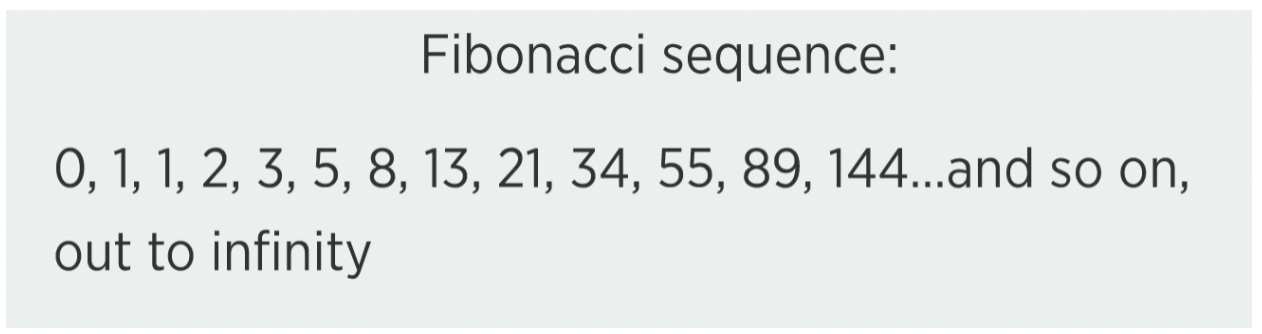As indicated by this sequence, we must begin with two "seed" integers, namely 0 and 1. After that, we add 0 and 1 to obtain the next digit in the series, which is 1. Then, you add that value to the previous one to obtain the next number in the sequence. If we continue along that path, we arrive at the following: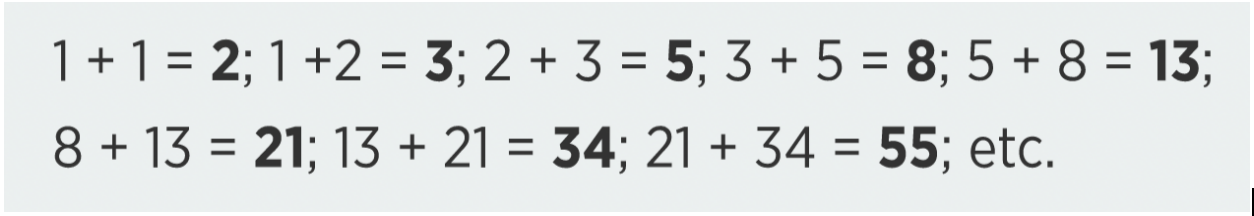The Fibonacci sequence is critical to our subject because it provides us with the numbers necessary to calculate our Fibonacci ratios. The Fibonacci ratios would not exist without the Fibonacci sequence.

## What Is a Fibonacci Ratio?

With the advent of the internet, there has been a great deal of confusion over the values that comprise Fibonacci Ratios. The widespread use of Fibonacci analysis, particularly in the domain of trading, has bred misconceptions and misunderstandings about how and what constitutes a Fibonacci ratio. Consider what a Fibonacci ratio is, how it is calculated, and some instances of non-Fibonacci ratios.

## Fibonacci Ratios

The mathematics underlying the Fibonacci ratios is rather straightforward. All we have to do is choose a few numbers from the Fibonacci sequence and divide them evenly. Consider the following example: Take a number in the sequence and divide it by the number immediately following it.

0 ÷ 1 = 0

1 ÷ 1 = 1

1 ÷ 2 = 0.5

2 ÷ 3 = 0.67

3 ÷ 5 = 0.6

5 ÷ 8 = 0.625

8 ÷ 13 = 0.615

13 ÷ 21 = 0.619

21 ÷ 34 = 0.618

34 ÷ 55 = 0.618

55 ÷ 89 = 0.618

Have you seen a trend emerging here? From 21 to 34 divided by 34, you will ALWAYS get 0.618!

We could perform the same thing with any other number in the Fibonacci sequence. For instance, by dividing a number in the series by the preceding number, another constant number forms.

1 ÷ 0 = 0

1 ÷ 1 = 1

2 ÷ 1 = 2

3 ÷ 2 = 1.5

5 ÷ 3 = 1.67

8 ÷ 5 = 1.6

13 ÷ 8 = 1.625

21 ÷ 13 = 1.615

34 ÷ 21 = 1.619

55 ÷ 34 = 1.618

89 ÷ 55 = 1.618

144 ÷ 89 = 1.618

Another pattern emerges from the Fibonacci numbers. Now, 1.618 is much more significant since it is also known as the Golden Ratio, the Golden Number, or the Divine Ratio, although I could go on and on about that subject for many more pages.

Here are some further instances of patterns that emerge by splitting the Fibonacci sequence's numbers in a pattern with other sequence numbers.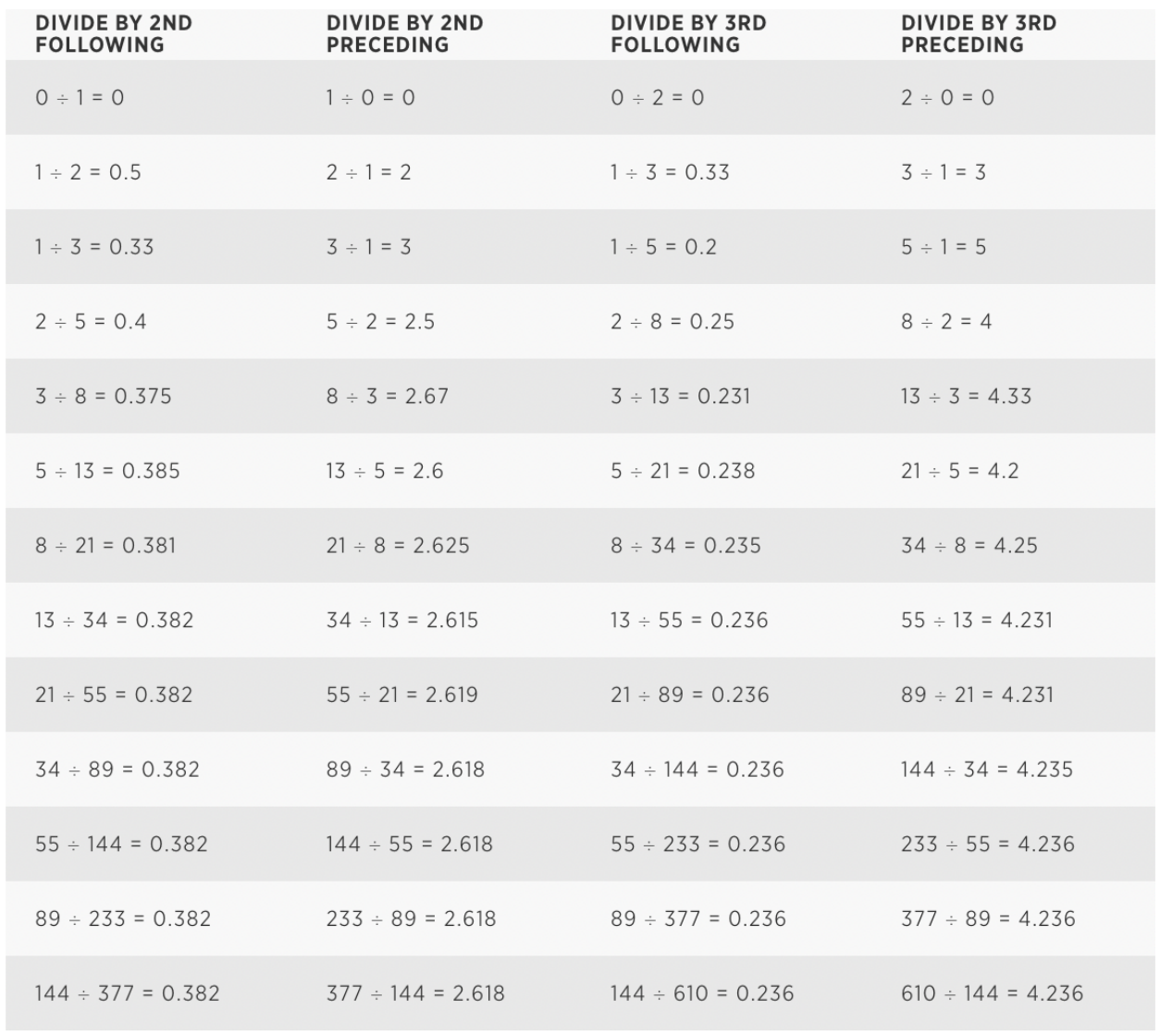As you can see, we may obtain a large number of distinct numbers just by choosing integers from the Fibonacci sequence and building a divisory pattern inside it. This is not, however, the sole method for calculating Fibonacci ratios. Once we get the division results, we may take the square roots of each of those numbers to obtain further numbers. The graphic below illustrates some of those values.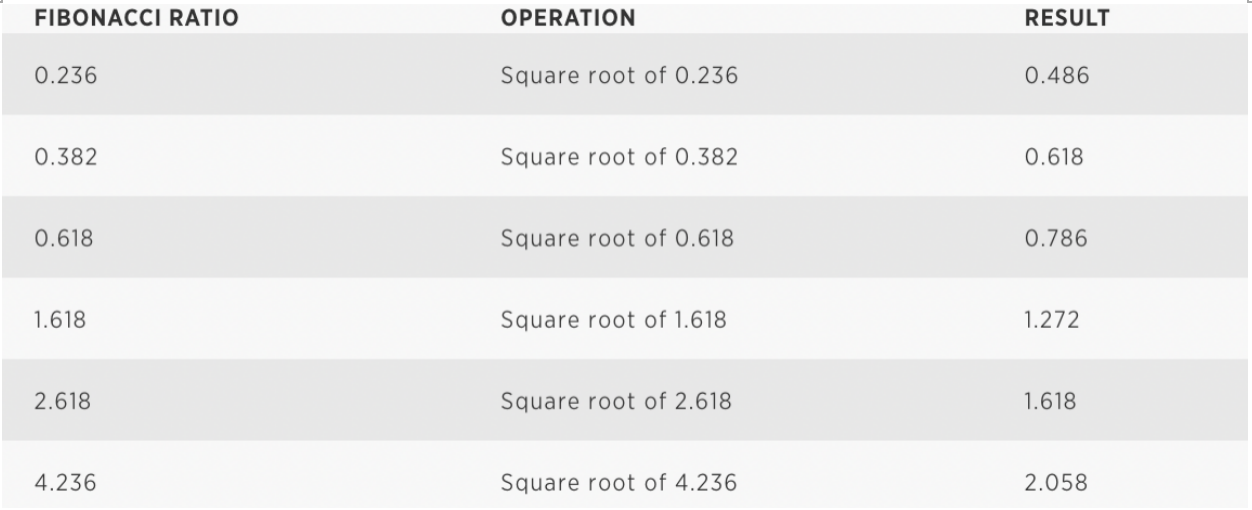The final step in converting these values to Fibonacci ratios is to convert them to percentages. 0.236 becomes 23.6 percent, 0.382 becomes 38.2 percent, and so on. Therefore, based on our investigation, 23.6 percent, 38.2 percent, 48.6 percent, 61.8 percent, 78.6 percent, 127.2 percent, 161.8 percent, 205.8 percent, 261.8 percent, and 423.6 percent are our genuine Fibonacci ratios.

While it is frequently employed in Fibonacci analysis, the 50% ratio is not a Fibonacci ratio. According to some, the 50% level is a Gann ratio developed in the early 1900's by W.D. Gann. Others refer to the 50% level as the opposite of a "holy ratio." Similar to the Fibonacci ratios, many people use the inverse or square root of the "holy ratios" to generate additional values. The table below has several instances.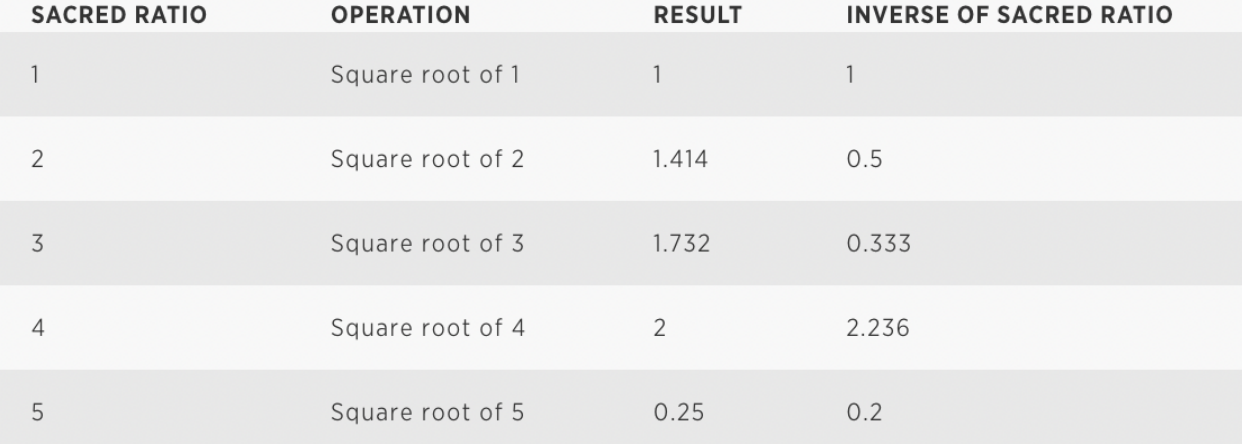Whichever source is used, the 50% ratio appears to be a very significant and meaningful level while trading, and hence is frequently included in Fibonacci analysis as if it were a Fibonacci ratio. Several additional values in the table have been misidentified as Fibonacci ratios, but are clearly not.

Whichever source is used, the 50% ratio appears to be a very significant and meaningful level while trading, and hence is frequently included in Fibonacci analysis as if it were a Fibonacci ratio. Several additional values in the table have been misidentified as Fibonacci ratios, but are clearly not.